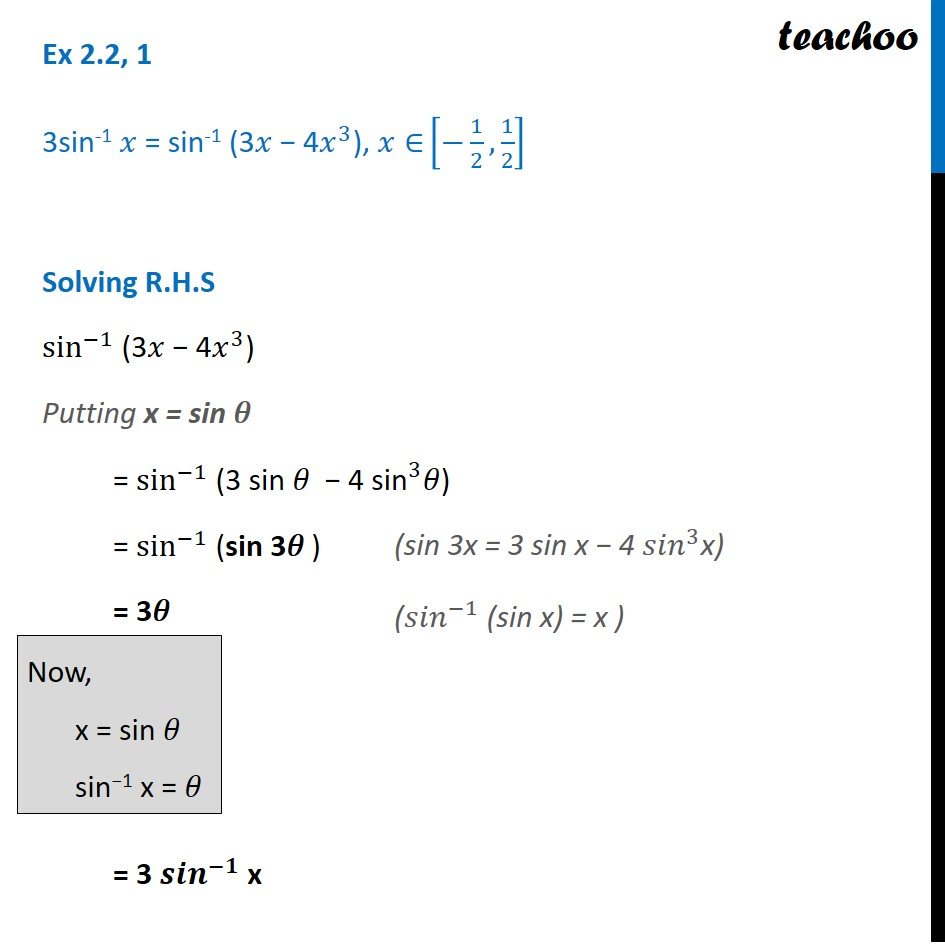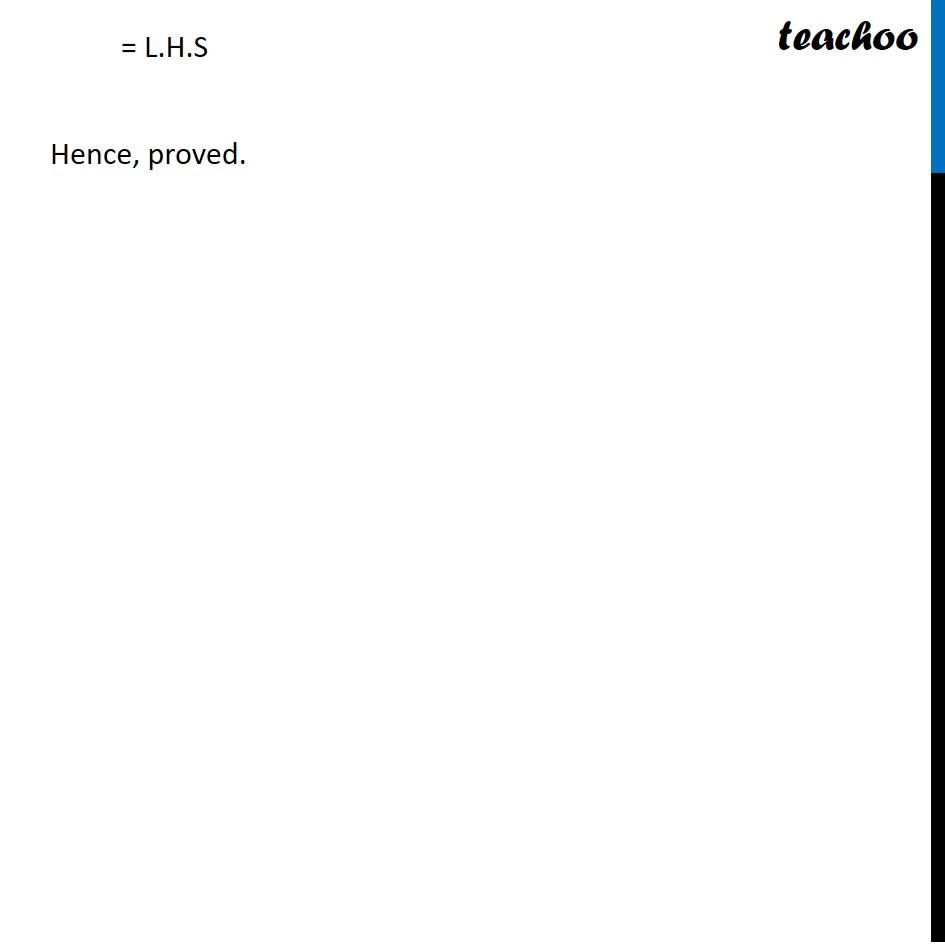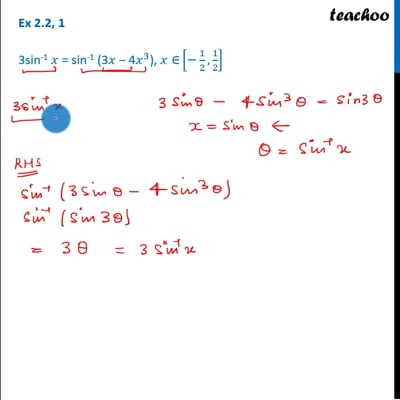Formulae based

Chapter 2 Class 12 Inverse Trigonometric Functions
Concept wiseThis video is only available for Teachoo black users

Solve all your doubts with Teachoo Black (new monthly pack available now!)

### Transcript

Ex 2.2, 1 3sin-1 𝑥 = sin-1 (3𝑥 − 4𝑥^3), 𝑥∈ [−1/2,1/2] Solving R.H.S sin^(−1) (3𝑥 − 4𝑥^3) Putting x = sin 𝜃 = sin^(−1) (3 sin 𝜃 − 4 〖"sin" 〗^3𝜃) = sin^(−1) (sin 3𝜃 ) = 3𝜃 = 3 〖𝒔𝒊𝒏〗^(−𝟏) x Now, x = sin 𝜃 sin−1 x = 𝜃 (sin 3x = 3 sin x − 4 〖𝑠𝑖𝑛〗^3x) (〖𝑠𝑖𝑛〗^(−1) (sin x) = x ) = L.H.S Hence, proved.